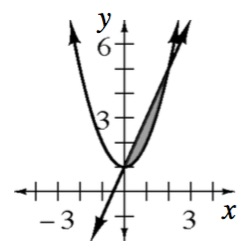### Home > APCALC > Chapter 8 > Lesson 8.4.1 > Problem8-142

8-142.

The region $M$ is enclosed by the functions $f(x) = x^2 + 1$ and $g(x) = 2x + 1$. Set up, but do not evaluate, the integrals to represent the volumes of the solids formed by revolving $M$ about each of the following axes.1. The $x$-axis.

Sketch the axis of rotation (flag pole) then use washers.

$V=\pi \int_{x=a}^{x=b}(R)^{2}-(r)^{2}dx$

1. The $y$-axis.

Use shells.

$V=2\pi \int_{x=a}^{x=b}xf(x)dx$

Note the $x$ represents the length of the radius, it's part of the $\text{base} = 2πr$ equation. And $f(x)$ represents the height of each shell.

1. The line $y = 5$.

Refer to the hint in part (a).

Recall that $R$ and $r$ represent radii of an outer and inner solid (the 'inner' solid is the hole). Make $R$ the function with the longer radii and $r$ the function with the shorter radii. Note: It is possible that $R$ is below $r$ on the graph. Once rotation happens, this will switch.

Also note that both radii have different lengths than $f(x)$ and $g(x)$, since the axis of rotation is $5$ units above the $x$-axis. Adjust the integral in part (a) accordingly:

$V=\pi \int_{x=a}^{x=b}(k-R)^{2}-(k-r)^{2}dx$

1. The line $x = 4$.

As in part (b), to avoid using horizontal rectangles (and rewriting the integrand in terms of $y$), you can use shells.

The setup should look exactly like the setup in part (b), with one exception: The radii are no longer the same values as the bounds. They will be either longer or shorter than the bounds. So shift the radii $k$ units to the left or right:

$V=2\pi \int_{x=a}^{x=b}(x-k)f(x)dx$